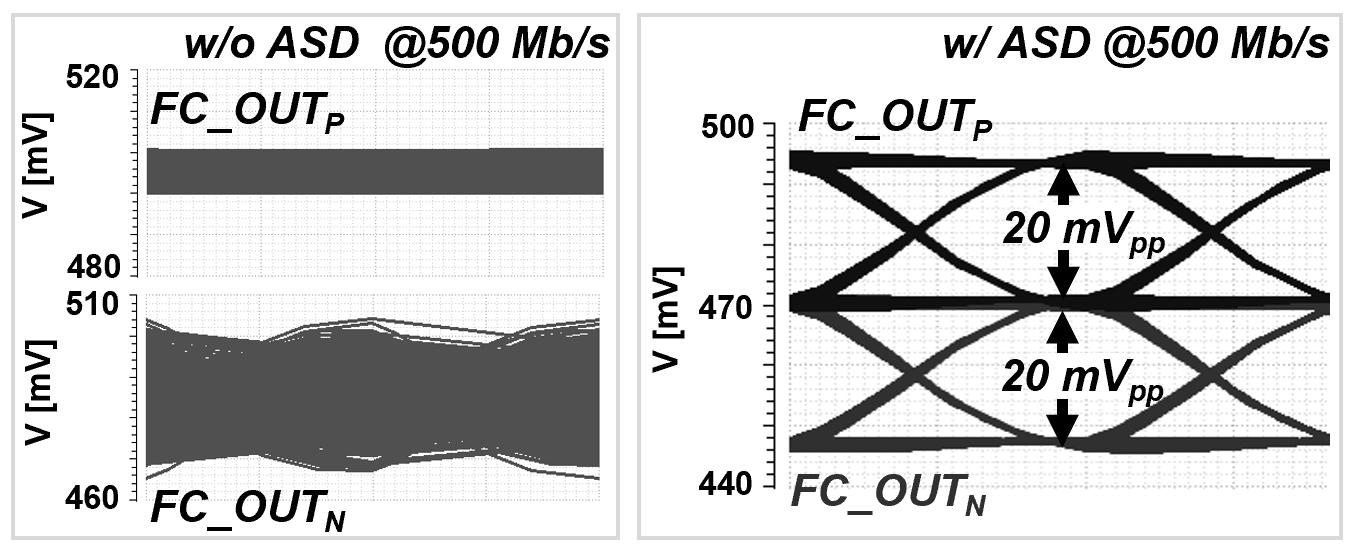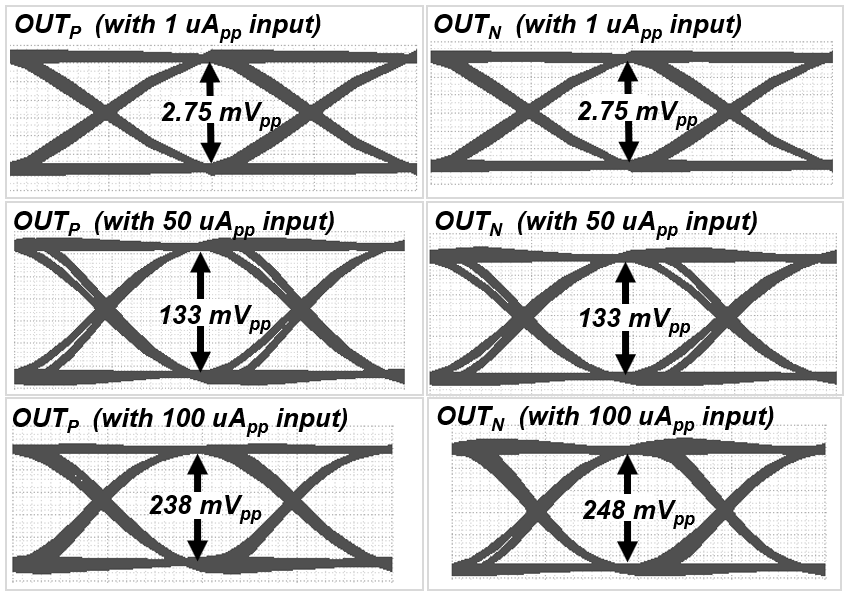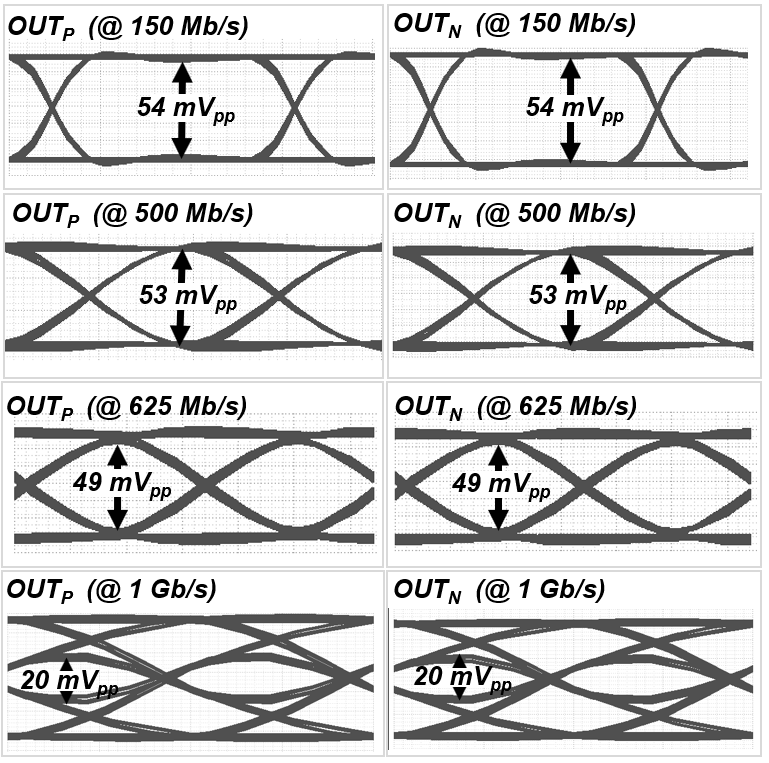Mobile QR Code1. (Dept. of Electronic and Electrical Eng., Ewha Womans University)

active single-to-differential, CMOS, dual-feedback, folded-cascode, TIA

## I. INTRODUCTION

Recently, light detection and ranging (LiDAR) sensors have been proliferated by the development of various industrial applications including autonomous vehicles, 5G communications, Internet of things (IoT), robotics, near-field image processing, etc (1). Especially, LiDAR sensors would be widely exploited even in smart factories and for home monitoring elder-care systems, because they can provide advantages over conventional RF radar sensors such as negligible signal interference, fast lock-on time, small beam spread, and better ability to track decelerating targets (2).

Fig. 1 shows the block diagram of a typical LiDAR sensor that can achieve high image resolution and depth perception utilizing time-of-flight (ToF) pulses.

However, this ToF-based LiDAR sensor inherently shows a major limitation, i.e., severely deteriorated detection ability by weather conditions, which should therefore be resolved by improving transimpedance gain and noise characteristics of optical receivers (3).

As shown in Fig. 1, the laser source emits short optical pulses while the optical detector receives the reflected signals from targets. Optical detectors (i.e., typically photodiodes) convert the received optical pulses into weak electrical currents and therefore, the following front-end transimpedance amplifier (TIA) mandates high transimpedance gain and low noise characteristics simultaneously.

For this purpose, a single-ended shunt-feedback TIA architecture has been commonly employed (4). However, it is well known that the single-ended configuration is vulnerable to common-mode noises, such as power supply noise and crosstalk noise generated from silicon substrate. Previously, several differential TIAs were suggested to alleviate these issues (5-10). In particular, a fully differential structure is desirable even from the input stage in order to improve the required common-mode rejection ratio, e.g. power supply rejection ratio (PSRR). Hence, we have demonstrated a couple of fully differential TIAs (5,6). Yet, they showed poor PSRR performances because of the inevitable asymmetry occurred mainly from the AC-coupling capacitor integrated at the input stage.

Moreover, the cascode configuration needs a large supply voltage to accommodate the stacked transistors. In this paper, we propose a number of novel circuit techniques to alleviate all these design issues. First, a folded-cascode architecture is suggested to lower the supply voltage (11). Second, a dual-feedback is applied at the input stage to decrease the input impedance further, hence extending the bandwidth to facilitate the narrow-pulse recovery. Third, an active single-to-differential (ASD) stage is exploited to maintain the same common-mode voltage at differential output nodes, thus obtaining fully differential signaling even from the input stage.

In short, we present a novel dual-feedback folded-cascode fully differential transimpedance amplifier (DFD-TIA) in this paper. Fig. 2 shows the block diagram of the proposed DFD-TIA, which consists of a dual-feedback folded-cascode input stage, an ASD stage for fully differential signaling, a post amplifier (PA) for gain boosting, and an output buffer (OB) for 50-Ω impedance matching.

## II. PROPOSED DFD-TIA

As aforementioned, shunt-feedback voltage-mode TIAs have been very popular because of their low-noise characteristics. Among those, inverter-based TIAs have become very attractive for long-range LiDAR applications. However, there exists a notorious design tradeoff between gain and bandwidth in the inverter-based TIAs. We have previously demonstrated a novel voltage-mode CMOS feedforward TIA to alleviate the design tradeoff (12). Yet, it was a single-ended configuration, thus leading to poor PSRR characteristics. In this work, therefore, we propose a novel dual-feedback folded-cascode input configuration, which improves the PSRR efficiently.

### 1. Dual-Feedback Folded-Cascode Input Stage

Fig. 3 depicts the schematic diagram of the proposed differential dual-feedback folded-cascode input configuration that comprises eight transistors (M$_{1}$~M$_{8}$). Here, M$_{3}$ & M$_{4}$ function as cascode transistors to reduce the Miller effect of the common-source transistors (M$_{1}$ & M$_{2}$). Thereby, the noise performance of this input stage can be improved by increasing the size of M$_{1}$ & M$_{2}$ for a similar input capacitance. Besides, the folded-cascode topology facilitates the biasing of transistors and enables the usage of a low supply voltage.

Meanwhile, two negative feedbacks are applied in this configuration. First, a conventional negative feedback is applied from the output node to the input node via R$_{\mathrm{F2}}$. Second, another negative feedback is applied from the drains of M$_{1}$ & M$_{2}$ to the input node via R$_{\mathrm{F1}}$. This dual-feedback topology leads to a lower input resistance than the case of a conventional shunt-feedback TIA, hence moving the input-node pole to a higher frequency. Then, a rather large photodiode capacitance can be tolerated for a similar bandwidth.

Also, this dual-feedback architecture renders the input current signals (i$_{\mathrm{pd}}$) from the photodiode to reach the output node in two paths (13). First, the input current (i$_{\mathrm{pd}}$) generates a negative voltage signal (v$_{1}$) through the common-source transistor (M$_{1}$) with R$_{\mathrm{F1}}$, which is amplified to be FC_OUT$_{\mathrm{N}}$ by the cascode stage (M$_{3}$). Second, the same current is amplified by the core amplifier (M$_{1}$,M$_{3}$,M$_{5}$) again, so as to generate another FC_OUT$_{\mathrm{N}}$ voltage which will be added to the previous FC_OUT$_{\mathrm{N}}$.

According to small signal analysis, the input resistance (R$_{\mathrm{in}}$) and the transimpedance gain (Z$_{\mathrm{T}}$) of the dual-feedback folded-cascode input stage are given by,

##### (1)
$R_{in}=\frac{v_{in}}{i_{pd}}\cong \left(\frac{g_{m3{R_{F1}}}}{g_{m1}+g_{m3}}\right)||\left(\frac{R_{F2}}{1+g_{m1}r_{o5}}\right)$ $\cong \left(\frac{R_{F1}}{2}\right)||\left(\frac{R_{F2}}{g_{m1}r_{o5}}\right)$

##### (2)
$$Z_{T} \cong-\left[\left(\frac{g_{m_{3}}r_{05}}{2}\right) R_{F 1}+R_{F 2}\right]$$

, where it is assumed that g$_{\mathrm{m1}}$ = g$_{\mathrm{m3}}$ and that g$_{\mathrm{m1}}$r$_{\mathrm{o5}}$ is greater than unity.

It is clearly seen that the proposed dual-feedback folded-cascode input stage can provide much lower input impedance and twice higher transimpedance gain at least, when compared to a conventional inverter TIA. Yet, the feedback resistance (R$_{\mathrm{F1}}$ & R$_{\mathrm{F2}}$) should be judiciously selected to optimize the design tradeoff between the transimpedance gain and the bandwidth.

Nonetheless, the differential core amplifier (M$_{2}$,M$_{4}$,M$_{6}$) still generates a DC output voltage at the drain node of M$_{4}$ because no input current is applied to the gate of M$_{2}$. This will deteriorate the PSRR due to the asymmetric signaling. Therefore, active single-to-differential circuit is employed at the output of the dual-feedback folded-cascode input stage to recover a fully differential signaling. It is also noted that the capacitor (C$_{1}$) at the gate of M$_{2}$ mimics the photodiode capacitance (C$_{\mathrm{pd}}$) for symmetry further.

### 2. Active Single-to-Differential Stage

Fig. 4 shows the schematic diagram of the proposed ASD stage, where two differential pairs are cross-connected. M$_{9}$ & M$_{12}$ take the asymmetric differential outputs of the preceded input stage, and the drain node (V$_{\mathrm{ASD}}$) of M$_{13}$ is connected to the gate of M$_{5}$ & M$_{6}$ in the input stage. Thereby, the ASD stage can recover a positive output voltage (FC_OUT$_{\mathrm{P}}$) and achieve a fully differential signaling.

The mechanism of this differential swings can be described as below. With no input signals, the same bias current of I/2 flows through M$_{9}$~M$_{12}$, and the current sources M$_{13}$ & M$_{14}$ maintain the bias current of I. Assuming that the gate voltage of M$_{9}$ drops by ∆v$_{\mathrm{g}}$ with an AC output signal occurred from the preceded input stage, it will increase the drain current (i$_{\mathrm{D9}}$) by α. On the contrary, the gate of M$_{10}$ is tied up to a constant voltage (V$_{\mathrm{REF}}$) and M$_{13}$ should maintain the bias current. This indicates that the drain current of M$_{10}$ must be reduced the same amount of α by pulling down the source voltage of M$_{10}$.

By denoting the variations of the gate and the source voltages as ∆v$_{\mathrm{g}}$ and ∆v$_{\mathrm{s}}$, respectively, the drain currents of M$_{9}$ & M$_{10}$ are given by,

##### (3)
$$\begin{array}{l} \frac{1}{2}+\alpha=\frac{1}{2} k_{p}\left(v_{S G}-\left|v_{t p}\right|+\Delta v_{g}-\Delta v_{s}\right)^{2} \\ =\frac{1}{2} k_{p}\left(v_{o v}+\Delta v_{g}-\Delta v_{s}\right)^{2} \end{array}$$

##### (4)
$$\begin{array}{l} \frac{1}{2}-\alpha=\frac{1}{2} k_{p}\left(v_{S G}-\left|v_{t p}\right|-\Delta v_{s}\right)^{2} \\ =\frac{1}{2} k_{p}\left(v_{O V}-\Delta v_{s}\right)^{2} \end{array}$$

Rearranging (3) & (4) provides two equations below.

##### (5)
$$\alpha \cong k_{p} v_{o V}\left(\Delta v_{g}-\Delta v_{s}\right)$$

##### (6)
$$\alpha \cong k_{p} v_{o V} \Delta v_{s}$$

By equating these two equations,

##### (7)
$$\Delta v_{s} \cong \frac{1}{2} \Delta v_{g}$$

The same procedure is applied to M$_{11}$ & M$_{12}$, in which the drain current of M$_{11}$ certainly increases the same amount of α to keep the bias current of M$_{13}$ constant, whereas the drain current of M$_{12}$ decreases by α simultaneously. Thereby, the gate voltage of M$_{12}$ is forced to pull up to generate the same AC output voltage (∆v$_{\mathrm{g}}$) at the differential node of the preceded dual-feedback folded-cascode input stage. Fig. 5 compares the post-layout simulated differential output eye-diagrams of the dual-feedback folded-cascode input stage at 500 Mb/s. With the proposed ASD stage applied, it is clearly seen that two identical differential eyes are clearly obtained, confirming the potential to achieve better common-mode rejection ratio.

Fig. 5. Simulated differential output eye-diagrams of the DFD input stage without and with the ASD stage for 20 μA$_{\mathrm{pp}}$ 2$^{31}$-1 PRBS inputs at 500-Mb/s operations## III. SIMULATION RESULTS

Design of the proposed DFD-TIA was realized in a standard 65-nm CMOS technology, where the input optical detector, i.e. photodiode, was emulated by its electrical lumped-model with a 25 Ω series resistor and a 1.6 pF parasitic capacitance. Fig. 6 shows the core layout of the DFD-TIA, where the DFD input with ASD stage occupies the area of 0.007 mm$^{2}$, and the core occupies the area of 0.017 mm$^{\mathrm{2}}$.

Fig. 7 depicts the post-layout simulated frequency response of the DFD-TIA, revealing the transimpedance gain of 67 dBΩ and the bandwidth of 330 MHz. It achieves the average noise current spectral density of 10.2 pA/$\sqrt{\mathrm{Hz}}$ that corresponds to the estimated sensitivity of -26 dBm for the BER of 10$^{-12}$ with the assumption of 0.6-A/W photodiode responsivity. At the worst cases of corner simulations, the transimpedance gain reduces 0.5 dB and the noise current spectral density worsens 6 % only. Even though the bandwidth shrinks down to 270 MHz, we believe it would barely affect the front-end circuit of a LiDAR system. Fig. 8 compares the power supply rejection ratio (PSRR) of the DFD-TIA together with that of MCD-TIA in (5), showing the PSRR of less than -19 dB up to 1 GHz.

Fig. 9 depicts the post-layout simulated differential output eye-diagrams of the DFD-TIA at 500 Mb/s with different input currents of 1 μA$_{\mathrm{pp}}$, 50 μA$_{\mathrm{pp}}$, and 100 μA$_{\mathrm{pp}}$, respectively. The matched differential eyes are shown for the input currents less than 100 μA$_{\mathrm{pp}}$ (only 4-% mismatch), confirming the fully differential signaling.

In Fig. 10, the output signals provide wide and clean eye-openings for 20 μA$_{\mathrm{pp}}$ 2$^{31}$-1 PRBS inputs at different data rates of 150 Mb/s, 500 Mb/s, 625 Mb/s, and 1 Gb/s, respectively.

Fig. 9. Simulated differential eye-diagrams of the DFD-TIA for different 2$^{31}$-1 PRBS inputs at 500 Mb/sTable 1. Performance comparison with previously reported TIAs.

 Parameters (6) (9) (10) (12) this work CMOS technology (nm) 130 *350 180 180 65 Input configuration BFD RGC P_INV VCF DFD Topology Differential Single-ended Differential Single-ended Differential Supply voltage (V) 1.2 5.0 3.3 1.8 1.2 Photodiode cap. (pF) 0.5 1.5 1.0 0.5 1.6 TZ gain (dBΩ) 66 58.5 106 (w/ PA+OB) 76.3 (w/ PA+OB) 67 Bandwidth (MHz) 580 200 150 720 330 Noise current spectral density $(\mathrm{pA}/\sqrt{\mathrm{Hz}})$ 7.9 9.2 4.55 6.3 10.2 PSRR (dB) @ 100 kHz -23 - - - -77.2 **Sensitivity (dBm) @ BER = $10^{-12}$ -25.7 -27.3 -31 -26 -26 Power dissipation (mW) 4.5 220 (w/ PA+TDC) 165 (w/ PA+OB) 7.4 3.38

BFD: bootstrapped fully differential, RGC: regulated cascade-coupled, P_INV: pseudo inverter

VCF: voltage-mode CMOS feedforward, PA: post-amplifier, TDC: time-to-digital converter, OB: output buffer

*BiCMOS, **Estimated by assuming 0.6-A/W responsivity

Fig. 10. Simulated differential output eye-diagrams of the DFD-TIA with 20 μA$_{\mathrm{pp}}$ 2$^{31}$-1 PRBS inputs at different data rates of 150 Mb/s, 500 Mb/s, 625 Mb/s, and 1 Gb/s.Table I compares the performance of the proposed DFD-TIA with the previously reported TIAs. The BFD-TIA in (6) showed worse PSRR and 25 % larger power consumption than the proposed DFD-TIA for a similar sensitivity. The single-ended RGC TIA in (9) obtained 12.7 % lower transimpedance gain and 40 % narrower bandwidth with a smaller supply voltage than the DFD-TIA. Yet, the P_INV TIA in (10) showed better noise and transimpedance gain which were, however, mainly due not only to 55 % narrower bandwidth, but also to the following post-amplification. The VCF-TIA in (12) was severely vulnerable to common-mode noises due to its single-ended configuration.

## IV. CONCLUSIONS

We have presented a novel dual-feedback folded-cascode TIA with active single-to-differential stage for fully differential signaling even from the input stage, hence improving common-mode noise immunity. The DFD-TIA realized in a 65-nm CMOS process provides fully differential output eye-diagrams with -77.2-dB PSRR at 100 kHz, -26-dBm sensitivity, and 3.38-mW power dissipation from a single 1.2-V supply. Conclusively, the proposed DFD-TIA can provide a low-power noise-immune solution for the applications of LiDAR sensors.

### REFERENCES

1
Liu M., Liu H., Li X., Zhu Z., Mar 2020, A 60-m Range 6.16-mW Laser-Power Linear-Mode LiDAR System With Multiplex ADC/TDC in 65-nm CMOS, IEEE Tran. Circuits and Systems I, Vol. 67, No. 3, pp. 753-7642
Buchbinder E., , Speed Detection: LADAR, www.ee.eng.buffalo.edu/faculty/cartwright/teaching/ee494s99/presentations/lasar.pdf.3
Yoshioka K., Nov 2018, A 20-ch TDC/ADC hybrid arch. LiDAR SoC for 240X96 pixel 200-m range imaging with smart accumulation tech. and residue quantizing SAR ADC, IEEE J. of Solid-State Circuits, Vol. 53, No. 11, pp. 3026-30384
Kim J., Buckwalter J. F., Mar 2012, A 40-Gb/s Optical Transceiver Front-End in 45nm SOI CMOS, IEEE J. of Solid-State Circuits, Vol. 47, No. 3, pp. 615-6265
Kim S. G., May 2019, A 40-GHz Mirrored-Cascode Differential TIA in 65nm CMOS, IEEE J. of Solid-State Circuits, Vol. 54, No. 5, pp. 1468-14746
Park Y., Kim J. -H., Park S. M., Feb 2020, Bootstrapped fully differential CMOS transimpedance amplifier, J. of Semiconductor Tech. and Sci., Vol. 20, No. 1, pp. 1-77
Sackinger E., 2005, Broadband Circuits for Optical Fiber Communication, Ch. 5, Wiley, pp. 1388
Kurtti S., Nissinen J., Kostamovaara J., Mar 2017, A Wide Dynamic Range CMOS Laser Radar Receiver With a Time-Domain Walk Error Compensation Scheme, IEEE Tran. Circuits and Systems I, Vol. 64, No. 3, pp. 550-5619
Kurtti S., Kostamovaara J., Mar 2009, Laser radar receiver channel with timing detector on front end unipolar-to-bipolar pulse shaping, IEEE J. Solid-State Circuits, Vol. 44, No. 3, pp. 835-84710
Zheng H., Ma R., Liu M., Zhu Z., Nov 2018, A Linear Dynamic Range Receiver With Timing Discrimination for Pulsed TOF Imaging LADAR Application, IEEE Trans. Instrum. Meas., Vol. 67, No. 11, pp. 2684-269111
Lima E. N., Cura J. L., Alves L. N., 2013, Folded-cascode transimpedance amplifiers employing a CMOS inverter as input stage, European Conf. on Circuit Theory and Design (ECCTD) 2013, Dresden, pp. 1-412
Hong C., Kim S. -H., Kim J. -H., Park S. M., Sep 2018, A linear-mode LiDAR sensor using a multi-channel CMOS TIA array, IEEE Sensors J., Vol. 18, No. 17, pp. 7032-704013
Abd-Elrahman D., 2015, Low power transimpedance amplifier using current reuse with dual feedback, in Proc.of IEEE Int. Conf. Electronics, Circuits, and Systems (ICECS), Cairo, pp. 244-247## Author

Yoonji Park received the B.S. degree in electronics engineering from Ewha Womans University, Seoul, South Korea, in 2018.

She is currently working toward the M.S. degree at the same university.

Her current research interests include CMOS analog integrated circuits and architectures for optical interconnects and LiDAR systems.

Sung Min Park received the B.S. degree in electrical and electronic engineering from KAIST, Korea, in 1993.

He received the M.S. degree in electrical engineering from University College London, U.K., in 1994, and the Ph.D. degree in electrical and electronic engineering from Imperial College London, U.K., in May 2000.

In 2004, he joined the faculty of the Department of Electronics Engineering at Ewha Womans University, Seoul, Korea, where he is currently a Professor.

His research interests include high-speed analog/digital integrated circuit designs in submicron CMOS and SiGe HBT technologies for the applications of optical interconnects, silicon photonics, and RF communications.

Prof. Park has served on the technical program committees of a number of international conferences including ISSCC (2004–2009).